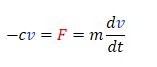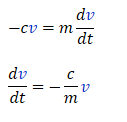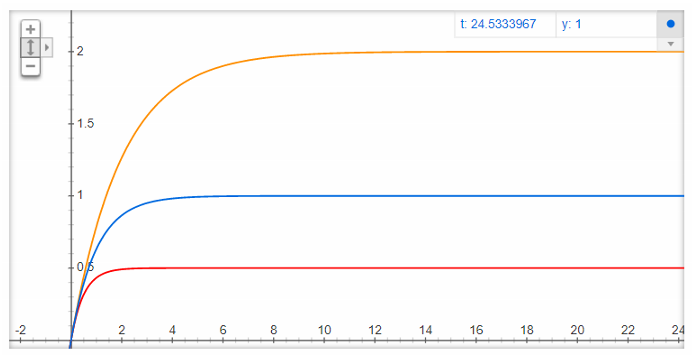top of page
Search

# Separation of variables tutorialKey concepts: Differential equations Separation of variables Speed and distance Question 1: "A car of mass, m, is moving at a speed v0 is put in neutral and gradually gets slowed down by an air resistance force that is some number times the speed of the car. Determine its speed as a function of time." To figure out this question, we need:

1. A sketch of the situation we are trying to describe

2. A differential equation that tells us how the change in speed depends on time and other variables

3. A strategy for solving this equation

4. A way of checking that the result makes sense

1. A sketch of the situation we are trying to describe

Let us make the sketch first and complete task 1. Initially:Looks good. Now for task 2.

2. A differential equation that tells us how the change in speed depends on time and other variables

We need some kind of equation that relates force to change in speed. Let us try with Newton's second lawHow big is the drag force? The question says that it is "some number times the speed", so let us write it as:^Notice that we need a negative sign to make sure that more forward speed means more drag force in the opposite direction. Obviously, the faster you run the more you will feel the air push back against you. We now have an interesting equation, that we can rewrite as:In plain English, this says: "How much the speed of the car changes with time is some negative number times its current speed!" Notice that the bigger the number c/m is, the more the speed decreases. We can make this number bigger by either increasing c or decreasing m. If we increase c, it means that the care becomes less aerodynamic. If we decrease m, it means that the car becomes lighter. Try tossing a fresh piece of paper through the air; it is broad and very light, so it will stop quickly. Anyways, enough beating around the bush. Let's see how to actually solve task 3.

3. A strategy for solving this equation

To solve the differential equation, we will use the strategy of "Separation of variables". The trick is to move all the factors that depend on v. To one side of the equality and all the things depending on t to the other side. In this case, we divide by v on both sides and multiply by dt:Nice. The next step is to integrate this expression on both sides. On the left, we integrate from the initial speed to the speed at some later time (from v0 to v(t)). On the right hand side, we integrate from t=0 to t=t:What do we do now? Let us remember that in the end, we want to find v(t). Therefore we can simply solve for it. Start by taking the exponential on both sides to get rid of the natural logarithm:Interesting! It seems that the speed decreases exponentially. How quickly the speed drops depends on c/m. Below, I have graphed the drop in speed for 3 cases: Yellow: c/m is small. The car is streamlined and heavy. Blue: c/m is somewhere in between the two cases above. Red: c/m is big. The car has bad aerodynamics and is light.As soon as we put the car in neutral, the speed starts to decrease. Initially the speed drops linearly, but later it drops more slowly. Let us not forget the final task. We need:

4. A way of checking that the result makes sense.

Have you ever been in a car or driven one? You probably know that if you release the gas pedal, the car does not immediately stop dead in its tracks. It can keep rolling for quite a while before it starts to slow down. This seems to match the gradual decrease in speed we see from the graphs. BONUS: That's right, you get to learn even more things than you expected! Let us try to figure out the total distance the car travels before it grinds to a halt. You probably remember that speed is the derivative of position with respect to time:We have the speed function, so let us integrate it to find the position as a function of time:Let us graph this! As before: Yellow: c/m is small. The car is streamlined and heavy. Blue: c/m is somewhere in between the two cases above. Red: c/m is big. The car has bad aerodynamics and is light.Looks like the car just travels "linearly" at first, but after a while it loses speed and stops at a certain distance. How do we find the total distance covered? We know that the more time passes, the more the speed drops towards zero. After a really long time, the speed will be exactly zero. When this happens, the traveled distance is:Does it make sense that the distance traveled is big, if the car is heavy and is initially going really fast? Does it make sense that if c is small (car is very aerodynamic) then it can roll for a long time? (Hint: Yes it does!) Question 2: "A space shuttle returns to earth and deploys a parachute to slow itself down as it lands on a runway. As the parachute unfolds, the drag force of the shuttle increases asDetermine the speed of the space shuttle as a function of time." As before, we need:

1. A sketch of the situation we are trying to describe

2. A differential equation that tells us how the change in speed depends on time and other variables

3. A strategy for solving this equation

4. A way of checking that the result makes sense

1. A sketch of the situation we are trying to describeYes, I know that is an almost photorealistic sketch of a space shuttle and no, you don't need to compliment my self-evident artistic skills. Anyways this sketch gives us some idea about how we expect the speed to change. Initially, the drag will be small, so the speed will not change much. As the parachute deploys and becomes more effective, the drag increases and the speed should drop more quickly. Now, let us solve 2.

2. A differential equation that tells us how the change in speed depends on time and other variables

We can re-use Newton's second law from Question 1. This time we will write it as:3. A strategy for solving this equation

To solve 3, we can once again apply separation of variables. We move all the things containing v to one side and everything with t to the other side. Then we integrate over speed and time. Finally, we solve for v(t):Let us try to graph this to see what is going on! Yellow: k/m is small. The shuttle is streamlined and heavy. Blue: k/m is somewhere in between the two cases above. Red: k/m is big. The shuttle has bad aerodynamics and is light.Now for the final part:

4. A way of checking that the result makes sense

By looking at the graphs, we can see that it takes a while before the speed starts to drop. This happens because t in Fd=ktv is small. The parachute has not deployed yet! As soon as it does, the speed begins to drop more and more quickly! That seems pretty sensible. BONUS: This time, let us try to determine the acceleration of the shuttle as a function of time. We can either take the derivative of v or simply reuse the result we got from Newton's second law: